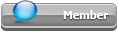0Hydrotest Pressure For Piping B31.3 - Significance Of Yield Strength

hydrotest pressure 1.5 times piping b31.3

3 replies to this topic
|

#1rs20170808@gmail.com

rs20170808@gmail.com

Veteran Member

•• Members
•• 41 posts

Posted 12 October 2019 - 02:15 PM

Hello all,

For hydrotest of piping from b31.3 the hydrotest pressure is 1.5 x design pressure x (stress value at test temperature/stress value at design temperature)

since the allowable stress value is does not exceed 2/3rd of the yield strength...does this formula for hydrotest incorporate this 2/3 rd factor?

is stress value at test temp. = 2/3 x yield strength at test temperature

is stress value at design temp. = 2/3 x yield strength at design temp.?

rs

#2fallah

fallah

Gold Member

•• ChE Plus Subscriber
•• 4,807 posts

Posted 13 October 2019 - 11:42 AM

For hydrotest of piping from b31.3 the hydrotest pressure is 1.5 x design pressure x (stress value at test temperature/stress value at design temperature)

since the allowable stress value is does not exceed 2/3rd of the yield strength...does this formula for hydrotest incorporate this 2/3 rd factor?

is stress value at test temp. = 2/3 x yield strength at test temperature

is stress value at design temp. = 2/3 x yield strength at design temp.?

Hi rs,

The allowable stresses in ASME B31.3 for each pressure rating have been specified based on 60% of yield strength at a temperature range, hence in the hydro test pressure of 1.5 x design pressure which is normally lower than (or in worst case equal to) the MAWP of relevant piping system would certainly create a maximum stress corresponding to 90% of the yield strength which leading to no problem in mechanical strength standpoint due to having adequate margin with reference to rated yield strength.

The correction for higher temperature is another story which come into play if it has to be considered.

#3rs20170808@gmail.com

rs20170808@gmail.com

Veteran Member

•• Members
•• 41 posts

Posted 13 October 2019 - 01:38 PM

For hydrotest of piping from b31.3 the hydrotest pressure is 1.5 x design pressure x (stress value at test temperature/stress value at design temperature)

since the allowable stress value is does not exceed 2/3rd of the yield strength...does this formula for hydrotest incorporate this 2/3 rd factor?

is stress value at test temp. = 2/3 x yield strength at test temperature

is stress value at design temp. = 2/3 x yield strength at design temp.?

Hi rs,

The allowable stresses in ASME B31.3 for each pressure rating have been specified based on 60% of yield strength at a temperature range, hence in the hydro test pressure of 1.5 x design pressure which is normally lower than (or in worst case equal to) the MAWP of relevant piping system would certainly create a maximum stress corresponding to 90% of the yield strength which leading to no problem in mechanical strength standpoint due to having adequate margin with reference to rated yield strength.

The correction for higher temperature is another story which come into play if it has to be considered.

Hello Fallah,

Thank you for clarification. Is this 60% of yield strength from b31.3? I could find tw0-thirds of yield strength only.

Is this some latest change in b31.3?

So this means that all the pipe thickness calculation formula from b31.3, the allowable stresses are based on 60% (or 2/3rd) of yield strength.

so when we go 1.5 times design pr. we are 1.5 x 0.6 = 0.9 so not more than 90% of yield strength.

#4fallah

fallah

Gold Member

•• ChE Plus Subscriber
•• 4,807 posts

Posted 13 October 2019 - 01:57 PM

Hello Fallah,

Thank you for clarification. Is this 60% of yield strength from b31.3? I could find tw0-thirds of yield strength only.

Is this some latest change in b31.3?

So this means that all the pipe thickness calculation formula from b31.3, the allowable stresses are based on 60% (or 2/3rd) of yield strength.

so when we go 1.5 times design pr. we are 1.5 x 0.6 = 0.9 so not more than 90% of yield strength.

Hi rs,

Not so difference between 60% and two-third...

The main intent is showing the concept that during the hydro test of a piping system the created stress shall not pass the limitation beyond it the pipe material can go to plastic deformation region...